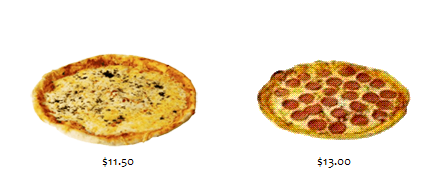← Back to X_TRADER® Help Library

#### Calculating Implied Ins

An Implied In price is generated by the differential of two contracts. The differential of our known values (the legs) goes into generating our unknown value (the spread).

Tip: The leg prices imply into the spread price.

Example: We have two variables in our example: a cheese pizza and a pepperoni pizza.Taking a look at our example, we know that...

• The price of the cheese pizza is \$11.50 and the price of the pepperoni pizza is \$13.00.
• Our unknown variable is the price differential between the two pizzas.
• The difference between the two pizzas is the pepperoni. This implies the cost of the pepperoni is \$1.50.

In this example, we have two known values (the cost of the cheese pizza and the cost of the pepperoni pizza). We need to calculate for a third value (i.e., the price differential).

Cheese Pizza

-

Pepperoni Pizza

=

Difference

\$11.50

-

\$13.00

=

\$1.50

Direct Price

Direct Price

Implied Price

(Outright)

(Outright)

The cost of the two known values implies the cost of the third value (i.e., the spread differential between the two legs).

Example: This example illustrates how to calculate an Implied In price for the implied Bid spread price.

To derive an implied spread Bid price, take the...

Leg 1April6000
Leg 2May 6011

Tip: Subtract the deferred contract from the near contract to generate the implied spread price.

6000

-

6011

=

x

x

=

-11

Taking a look at the market conditions in our example, we know that...

• We can sell April at 6000.
• We can buy May at 6011.
• This means that there is an implied bid in the April - May spread at -11.

Exchanges benefit when they disseminate implied prices. The exchange disseminates an implied -11 spread bid in the April - May spread to induce trades. If a trader hits the exchange's -11 bid, the matching engine would fill three orders:

• The sale of the April - May spread at -11 (Trader A)
• A bid in April at 6000 (Trader B)

By inducing a sale at -11, the matching engine fills three different orders, thus generating three different transactions. The exchange via the matching engine wants to fill the legs in the spread so it implies a bid in the spread. If the exchange implies a bid in the April – May spread, it can induce a trader to sell the spread at -11 (i.e., sell April at 6000 and buy May at 6011).

Example: This example illustrates how to calculate an Implied In price for the implied Ask spread price. Here again, the leg prices imply into the spread price.

Leg 1April 6001
Leg 2May6010

Tip: Subtract the deferred contract from the near contract to generate the implied spread price.

6001

-

6010

=

x

x

=

-9

Taking a look at the market conditions in our example, we know that...

• We can buy April at 6001.
• We can sell May at 6010.
• This means that there is an implied ask in the April - May spread at -9.